Test: The Root Locus Technique - 1

# Test: The Root Locus Technique - 1

Test Description

## 10 Questions MCQ Test GATE Electrical Engineering (EE) 2023 Mock Test Series | Test: The Root Locus Technique - 1

Test: The Root Locus Technique - 1 for Electronics and Communication Engineering (ECE) 2023 is part of GATE Electrical Engineering (EE) 2023 Mock Test Series preparation. The Test: The Root Locus Technique - 1 questions and answers have been prepared according to the Electronics and Communication Engineering (ECE) exam syllabus.The Test: The Root Locus Technique - 1 MCQs are made for Electronics and Communication Engineering (ECE) 2023 Exam. Find important definitions, questions, notes, meanings, examples, exercises, MCQs and online tests for Test: The Root Locus Technique - 1 below.
Solutions of Test: The Root Locus Technique - 1 questions in English are available as part of our GATE Electrical Engineering (EE) 2023 Mock Test Series for Electronics and Communication Engineering (ECE) & Test: The Root Locus Technique - 1 solutions in Hindi for GATE Electrical Engineering (EE) 2023 Mock Test Series course. Download more important topics, notes, lectures and mock test series for Electronics and Communication Engineering (ECE) Exam by signing up for free. Attempt Test: The Root Locus Technique - 1 | 10 questions in 30 minutes | Mock test for Electronics and Communication Engineering (ECE) preparation | Free important questions MCQ to study GATE Electrical Engineering (EE) 2023 Mock Test Series for Electronics and Communication Engineering (ECE) Exam | Download free PDF with solutions
 1 Crore+ students have signed up on EduRev. Have you?
Test: The Root Locus Technique - 1 - Question 1

### Which of the following option is correct? The root locus is the path of the roots of the characteristic equation traced out in the s-plane

Detailed Solution for Test: The Root Locus Technique - 1 - Question 1

The root locus is defined as location of closed loop poles when system gain k is varied from zero to infinity.

Test: The Root Locus Technique - 1 - Question 2

### Number of intersection of the asymptotes of the complete root loci is

Detailed Solution for Test: The Root Locus Technique - 1 - Question 2

Centroid is the intersection point of asymptotes of the complete root loci which lie on the real axis.

Test: The Root Locus Technique - 1 - Question 3

### The open-loop transfer function of a unity feedback control system is given by: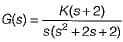The centroid and the angle of root locus asymptotes are respectively

Detailed Solution for Test: The Root Locus Technique - 1 - Question 3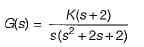Number of open loop poies = P = 3
and number of open loop zeros = Z = 1
Poles are at: s = -1 ± j, 0 and zero is at s = -2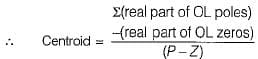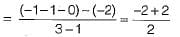= 0
Here, P - Z = 2
So, angle of asymptotes are given by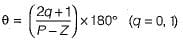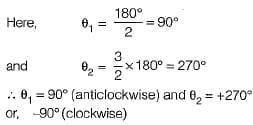Test: The Root Locus Technique - 1 - Question 4

Consider the loop transfer function K(s + 6) / (s + 3)(s +5 ) In the root locus diagram the centroid will be located at:

Detailed Solution for Test: The Root Locus Technique - 1 - Question 4

Centroid = Sum of real part of open loop pole-sum of real part of open loop zeros / P - Z.

Test: The Root Locus Technique - 1 - Question 5

Assertion (A): Root locus is a graphical method in which roots of the characteristic equation are plotted in s-plane for the different value of parameter.
Reason (R): The locus of the roots of the characteristic equation when gain is varied from zero to infinity is called root locus.

Test: The Root Locus Technique - 1 - Question 6

Assertion ( A ) : An addition of real pole at s = - p0 in the transfer function G(s)H(s) of a control system results in the increase of stability margin.
Reason ( R ) : An addition of real pole at s = - p0 in the transfer function G(s)H(s) will make the resultant root loci bend towards the right.

Detailed Solution for Test: The Root Locus Technique - 1 - Question 6

Addition of real pole to an open loop T.F. decreases the stability because root locus shifts towards right of s-plane. Hence, assertion is a false statement.

Test: The Root Locus Technique - 1 - Question 7

The open-loop transfer function of a closed loop control system is given as: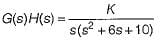Which of the following statements is correct about the root locus of the above system?

Detailed Solution for Test: The Root Locus Technique - 1 - Question 7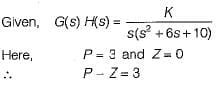Number of branches terminating at infinity = 3 = P - Z.
Number of branches terminating at zero = Z= 0.
Poles are at s = 0 and s = -3 ± j1.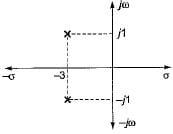Since, three number of poles have to terminate at infinity, therefore the two complex poles will terminate at infinity due to which there will be intersection of root locus branches with jω-axis. Now, characteristic equation is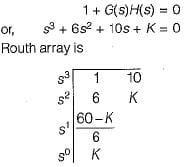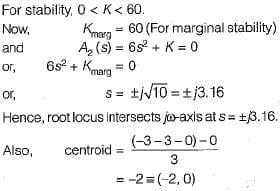Test: The Root Locus Technique - 1 - Question 8

The root locus of s(s - 1) + K(s + 1) = 0 is a circle. The co-ordinates of the centre and the radius (in units) of this circle are respectively

Detailed Solution for Test: The Root Locus Technique - 1 - Question 8

Given, s(s - 1) + K(s + 1) = 0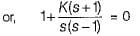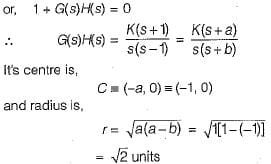Test: The Root Locus Technique - 1 - Question 9

What is the open-loop transfer function of a unity feedback control system having root locus shown in the following figure?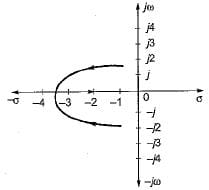Detailed Solution for Test: The Root Locus Technique - 1 - Question 9

Branches of root locus either terminate at infinity or zero. Here, one branch is terminating at s = -2, therefore there is a zero at s -2. Since two branches are meeting between s = -3 and s = -4, therefore there is a breakaway point between s = -3 and -4, Hence, there must be poles at s = (-1 ± j).
Therefore, open loop T.F. is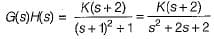Here, P = 2 and Z = 1.
Thus, one branch terminates at s = -2 (zero) and one branch terminate sat infinity ( P - Z = 1).

Test: The Root Locus Technique - 1 - Question 10

A control system has G(s)H(s)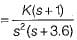(0 < K < ∞). The number of breakaway point in the root locus diagram is/are

Detailed Solution for Test: The Root Locus Technique - 1 - Question 10∴  Number of branches terminating at infinity = P - Z = 2.
Number of branches terminating at zero = Z = 1.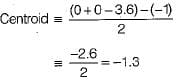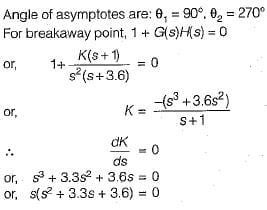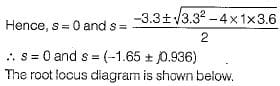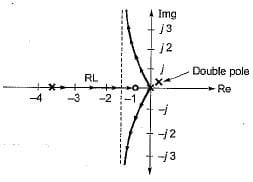Here, (-1.65 ± j0.936) is not a part of root locus. Thus, it has only one breakaway point s = 0.

## GATE Electrical Engineering (EE) 2023 Mock Test Series

21 docs|271 tests
 Use Code STAYHOME200 and get INR 200 additional OFF Use Coupon Code
Information about Test: The Root Locus Technique - 1 Page
In this test you can find the Exam questions for Test: The Root Locus Technique - 1 solved & explained in the simplest way possible. Besides giving Questions and answers for Test: The Root Locus Technique - 1, EduRev gives you an ample number of Online tests for practice

## GATE Electrical Engineering (EE) 2023 Mock Test Series

21 docs|271 tests